Function Repository Resource:

# StirlingsFormula

Compute an approximation to a factorial with Stirling's formula

Contributed by: Peter Cullen Burbery
 ResourceFunction["StirlingsFormula"][n] computes an approximation to n! with Stirling’s formula.

## Examples

### Basic Examples (4)

Compute an approximation for 5!:

 In:=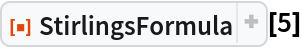Out=In:=Out=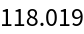Compute the absolute error by comparing to 5!:

 In:=Out=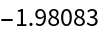Compute the relative error:

 In:=Out=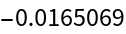Compute the relative error for 2018!:

 In:=Out=### Properties and Relations (1)

The formula can be computed with DiscreteAsymptotic:

 In:=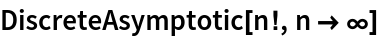Out=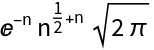Peter Burbery

## Version History

• 1.0.0 – 30 August 2022